# New Pattern Quantitative Aptitude Questions Based on IBPS PO Mains 2018 (Day-01)

Dear Aspirants, Quantitative Aptitude plays a crucial role in Banking and all other competitive exams. To enrich your preparation, here we have provided New Pattern Aptitude Questions that are similar to the questions asked in IBPS PO Mains 2018. Candidates those who are going to appear in upcoming bank exams like IBPS Clerk, IBPS SO, etc., can practice these questions daily and make your preparation effective.

[WpProQuiz 4440]

The series-I satisfy a certain pattern, follow the same pattern in Series-II and answer the questions given below.

1)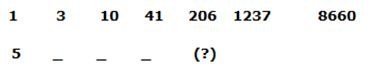a) 460

b) 406

c) 686

d) 866

e) 506

2)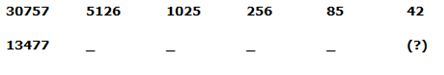a) 24

b) 36

c) 32

d) 18

e) 46

3)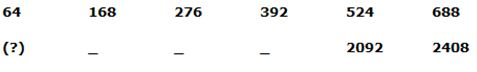a) 961

b) 1024

c) 512

d) 125

e) 343

4)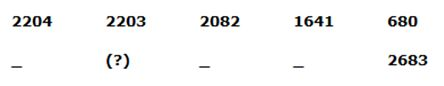a) 4207

b) 4205

c) 4208

d) 4209

e) 4206

5)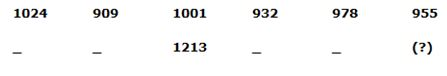a) 1144

b) 1121

c) 1236

d) 1190

e) 1167

6) Prem started the business with an investment of Rs.______. After three months, Kavin joined the business and invested Rs. 3800. At the end of the year the total profit earned is Rs. 35000 and the Kavin’s share is Rs._____.

Which of the following satisfies the two blanks given in the questions?

a) Rs.2400, Rs.19000

b) Rs.3600, Rs.18000

c) Rs.2800, Rs.17000

d) Rs.3200, Rs.17000

e) None of these

7) The distance between the station A and B is ______ km. A train leaves station A for station B and at the same time another train leaves station B for station A. Both the trains meet 11 hours after they start moving. If the train that starts from station A is 28 kmph faster than the other one and the ratio of the speeds of both the trains is ___:___.

Which of the following satisfies the two blanks given in the questions?

a) 583, 4:3

b) 924, 2:1

c) 858, 7:5

d) 759, 4:3

e) None of these

8) A boat can travel 21km upstream in 70 minutes. If the ratio of the speed of the boat in still water to the speed of the stream is 7:1. The boat takes _____ hours to cover ____km downstream.

Which of the following satisfies the two blanks given in the questions?

a) 4, 48

b) 3, 72

c) 5, 96

d) 2, 60

e) None of these

9) The train crosses the platform of length _____m and the pole in 24 seconds and 12 seconds respectively. The train crosses the man running in opposite direction with speed of _____kmph in 9 seconds.

Which of the following satisfies the two blanks given in the questions?

a) 400, 20

b) 300, 18

c) 200, 20

d) 180, 20

e) None of these

10) A and B entered into the business, A invested Rs. 2550 and B invested Rs.____. After 4 months, A double his investment for next 6 months then withdrew its investment. If they got total profit of Rs.12200 after a year and A’s profit share is Rs.____.

Which of the following satisfies the two blanks given in the questions?

a) Rs.2700, Rs.6800

b) Rs.3000, Rs.6400

c) Rs.3200, Rs.7000

d) Rs.2500, Rs.6400

e) None of these

Directions (1-5):

Series I pattern:

1 * 2 +1 = 3

3 * 3 + 1 = 10

10 * 4 +1 = 41

41 * 5 +1 = 206

206 * 6 +1 = 1237

1237 * 7 +1 = 8660

Series II pattern:

5 * 2 + 1 = 11

11 * 3 + 1 = 34

34 * 4 +1 = 137

137 * 5 +1 = 686

686 * 6 +1 = 4117

4117 * 7 +1 = 28820

Series I pattern:

30757 – 1 ÷ 6 = 5126

5126 – 1 ÷ 5 = 1025

1025 – 1 ÷ 4 = 256

256 – 1 ÷ 3 = 85

85 – 1 ÷ 2 = 42

Series II pattern:

13477 – 1 ÷ 6 = 2246

2246 – 1 ÷ 5 = 449

449 – 1 ÷ 4 = 112

112– 1 ÷ 3 = 37

37 – 1 ÷ 2 = 18

Series I pattern: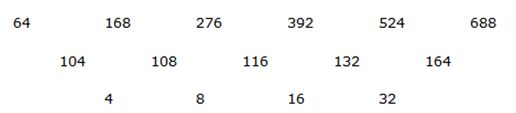Series II pattern: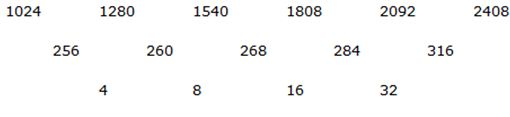Series I pattern:

2204 – 12 = 2203

2203 – 112 = 2082

2082 – 212 = 1641

1641 – 312 = 680

Series II pattern:

4207 – 12 = 4206

4206 – 112 = 4085

4085 – 212 = 3644

3644 – 312 = 2683

Series I pattern: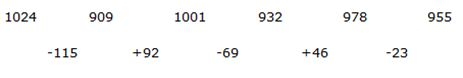Series II pattern: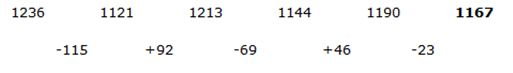From Option (A)

Profit ratio of Prem and Kavin=2400*12 : 3800*9

=16:19

Share of Kavin= (19/35)*35000 = Rs.19000

This satisfies the given condition.

From Option (B)

Profit ratio of Prem and Kavin=3600*12: 3800*9

=24:19

Share of Kavin= (19/43)*35000 = 15465.11

This not satisfy the given condition.

From Option (C)

Profit ratio of Prem and Kavin=2800*12 : 3800*9

=56:57

Share of Kavin=(57/113)*35000=17654.8

This not satisfies the given condition.

From Option (D)

Profit ratio Prem and Kavin=3200*12 : 3800*9

=64:57

Share of Kavin=(57/121)*35000=16487.6033

This not satisfies the given condition.

Let Speed of train A=x

Speed of train B=y

X-y=28

From Option (A)

x+y=583/11

x+y=53

2x=81

X=40.5

Y=12.5

Ratio=40.5:12.5=81:25

This not satisfies the given condition.

From Option (B)

x-y =28kmph

x+y=924/11=84

2x=112

X=56

Y=56-28=28

Ratio=56:28=2:1

This satisfies the given condition.

From Option (C)

x-y =28

x+y=858/11=78

2x=106

X=53

Y=25

Ratio=53:25

This not satisfies the given condition.

From Option (D)

x-y =28

x+y=759/11=69

2x=97

X=48.5

Y=20.5

Ratio=48.5:20.5=97:41

This not satisfies the given condition.

Speed of the boat=7x

Speed of the stream=x

Upstream speed = (7x-x) =6x

Downstream speed = (7x+x) =8x

Upstream speed, 6x=21*60/70

6x=18kmph

X=3kmph

Downstream speed=8(3) =24kmph

From Option (A)

Time taken=48/24=2 hr

This not satisfies the given condition.

From Option (B)

Time=72/24=3 hr

This satisfies the given condition.

From Option(C)

Time=96/24=4 hr

This not satisfies the given condition.

From Option (D)

Time=60/24=2.5 hr

This not satisfies the given condition.

Length of the train=x

Option (A)

Length of the platform = 400 m

Train crosses a pole and platform in 24 and 12 seconds respectively,

(x+400)/24 = x/12

X+400 = 2x

X=400 m

Speed of the train = 400/12 = 100/3 m/s

Train crosses a man and man’s speed x km/hr

400/(100/3 +z) = 9

400 = 900/3 +9z

300/3 = 9z

Z= 100/9 = 100/9 * 18/5 = 40 km/hr

This option is not satisfies the given condition.

From Option (B)

Length of the platform = 300 m

Train crosses a pole and platform in 24 and 12 seconds respectively,

(x+300)/24 = x/12

X+300 = 2x

X=300 m

Speed of the train = 300/12 = 25 m/s

Train crosses a man and man’s speed x km/hr

300/(25 +z) = 9

300 = 225 +9z

75 = 9z

Z= 75/9 = 75/9 * 18/5 = 30 km/hr

This option is not satisfies the given condition.

From option(C)

Length of the platform = 200 m

Train crosses a pole and platform in 24 and 12 seconds respectively,

(x+200)/24 = x/12

X+200 = 2x

X=200 m

Speed of the train = 200/12 = 50/3 m/s

Train crosses a man and man’s speed x km/hr

200/(50/3 +z) = 9

200 = 450/3 +9z

150/3 = 9z

Z= 50/9 = 50/9 * 18/5 = 20 km/hr

This option satisfies the given condition.

From Option (D)

Length of the platform = 180 m

Train crosses a pole and platform in 24 and 12 seconds respectively,

(x+180)/24 = x/12

X+180 = 2x

X=180 m

Speed of the train = 180/12 = 15 m/s

Train crosses a man and man’s speed x km/hr

180/(15 +z) = 9

180 = 135 +9z

45 = 9z

Z= 45/9 = 45/9 * 18/5 = 18 km/hr

This option is not satisfies the given condition.

From Option (A)

Profit ratio= (2550*4 + 5100*6) : (2700*12)

=34:27

A’s share= (34/61)*12200= 6800

This is satisfies the given condition.

From Option (B)

Profit ratio = (2550*4 + 5100*6) : (3000*12)

=17:15

A’s Share= (17/32)*12200=6481.25

This is not satisfies the given condition.

From Option (C)

Profit ratio= (2550*4 + 5100*6): (3200*12) = 17:16

A’s share = (17/33)*12200=6284.84

This is not satisfies the given condition.

From Option (D)

Profit ratio= (2550*4 + 5100*6):(2500*12)=34:25

A’s share=34/59*12200=7030.5

This is not satisfies the given condition.